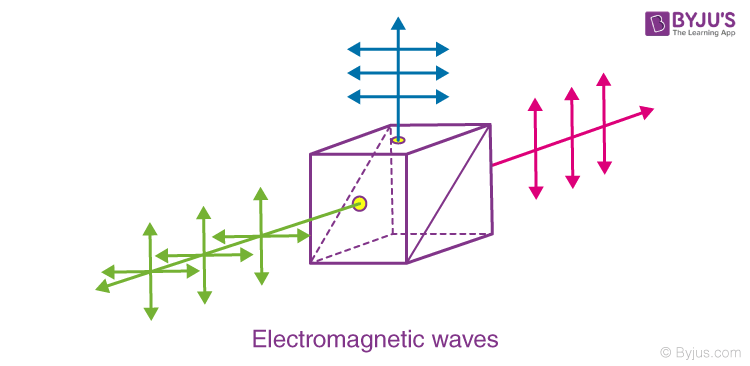# Transverse Nature of Electromagnetic Waves

In an electromagnetic wave, electric and magnetic field vectors are perpendicular to each other and at the same time are perpendicular to the direction of propagation of the wave. This nature of electromagnetic wave is known as Transverse nature.

Maxwell proved that both the electric and magnetic fields are perpendicular to each other in the direction of wave propagation. He considered an electromagnetic wave propagating along positive x-axis. When a rectangular parallelepiped was placed parallel to the three co-ordinate axis, the electric and magnetic fields propagate sinusoidal with the x-axis and are independent of y and z-axis.

The figure shows a rectangular parallelepiped in a positive direction.The rectangular parallelepiped does not enclose any charge thus the total electric flux across it must be zero. This law is called gauss’ law, i.e.

$$\begin{array}{l} \oint \overrightarrow{E}. \overrightarrow{ds} \end{array}$$
=0

Have you ever anchored one end of a rope and held the other end in your hand? You can create a transverse wave by moving your hands in a different direction.

The electric field remains the same at different points on the x-axis; this proves that the electric field is static in nature. It is known that static fields can propagate as a wave, hence EX = 0.

The component of the electric field along the direction of propagation is zero as the electric field is perpendicular to the direction of wave propagation.

This also proves for a magnetic field, as the magnetic field is also perpendicular to the direction of wave propagation. This proves the transverse nature of electromagnetic wave to the direction of wave propagation.

Stay tuned with BYJU’S for more such interesting articles. Also, register to “BYJU’S – The Learning App” for loads of interactive, engaging Physics-related videos and an unlimited academic assist.

Test Your Knowledge On Electromagnetic Waves Transverse Nature!